Download Presentation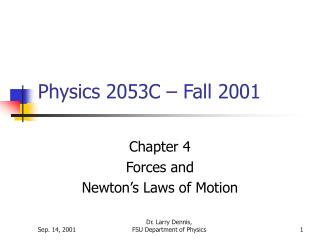Physics 2053C – Fall 2001

# Physics 2053C – Fall 2001 - PowerPoint PPT Presentation

Physics 2053C – Fall 2001. Chapter 4 Forces and Newton’s Laws of Motion. Forces. Newton’s Laws: If the force on an object is zero, then it’s velocity is constant. The acceleration = net force / mass.I am the owner, or an agent authorized to act on behalf of the owner, of the copyrighted work described.
Download Presentation## Physics 2053C – Fall 2001

An Image/Link below is provided (as is) to download presentation

Download Policy: Content on the Website is provided to you AS IS for your information and personal use and may not be sold / licensed / shared on other websites without getting consent from its author.While downloading, if for some reason you are not able to download a presentation, the publisher may have deleted the file from their server.

- - - - - - - - - - - - - - - - - - - - - - - - - - E N D - - - - - - - - - - - - - - - - - - - - - - - - - -
Presentation Transcript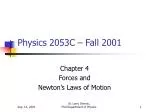### Physics 2053C – Fall 2001

Chapter 4

Forces and

Newton’s Laws of Motion

Dr. Larry Dennis,

FSU Department of Physics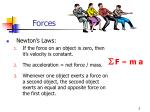Forces
• Newton’s Laws:
• If the force on an object is zero, then it’s velocity is constant.
• The acceleration = net force / mass.
• Whenever one object exerts a force on a second object, the second object exerts an equal and opposite force on the first object.

F = m a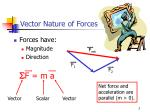Fnet

F1

F2

Vector Nature of Forces
• Forces have:
• Magnitude
• Direction

F = m a

Net force and

acceleration are

parallel (m > 0).

Vector

Scalar

Vector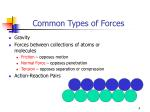Common Types of Forces
• Gravity
• Forces between collections of atoms or molecules
• Friction – opposes motion
• Normal Force – opposes penetration
• Tension – opposes separation or compression
• Action-Reaction Pairs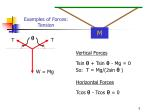Examples of Forces: Tension

M

T

T

Vertical Forces

Tsin  + Tsin- Mg = 0

So: T = Mg/(2sin  )

W = Mg

Horizontal Forces

Tcos  - Tcos= 0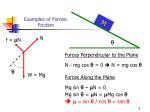M

Examples of Forces: Friction

N

f = N

Forces Perpendicular to the Plane

N - mg cos  = 0  N = mg cos 

W = Mg

Forces Along the Plane

Mg sin  - N = 0

Mg sin  = N = Mg cos 

  = sin  /cos  = tan 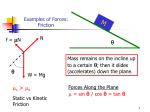M

Examples of Forces: Friction

N

f = N

Mass remains on the incline up

to a certain ; then it slides

(accelerates) down the plane.

W = Mg

s > k

Static vs Kinetic

Friction

Forces Along the Plane

 = sin  /cos  = tan 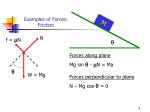M

Examples of Forces: Friction

N

f = N

Forces along plane

Mg sin  - N = Ma

Forces perpendicular to plane

N – Mg cos  = 0

W = Mg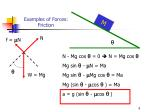M

Examples of Forces: Friction

N

f = N

N - Mg cos  = 0  N = Mg cos 

Mg sin  - N = Ma

Mg sin  - Mg cos  = Ma

Mg (sin  - cos  ) = Ma

a = g (sin  - cos  )

W = Mg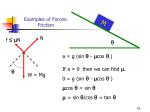M

Examples of Forces: Friction

N

f N

a = g (sin  - cos  )

If a = 0 then we can find .

0 = g (sin  - cos  )

cos  =sin 

 =sin /cos  = tan

W = MgTop Block

Bottom Block

Nt

Nb

T

f = Nt

mtg

f = Nt

Nt

mbg

T = 36 N

 = 0.4

Examples of Forces & Acceleration

1 kg

 = 20o

3 kg

aTop Block

Nt

f Nt

F = ma

mtg

T = 36 N

 = 0.4

Examples of Forces & Acceleration

1 kg

 = 20o

3 kg

a

Vertical Forces

Nt – mt g = may = 0

 Nt = mt g

Horizontal Forces

f = mt axF = ma

T = 36 N

 = 0.4

Examples of Forces & Acceleration

1 kg

 = 20o

mb = 3 kg

a

Bottom Block

Nb

T

f = Nt

Vertical Forces

Nb + T sin – mbg - Nt = mbay = 0

 Nb = mbg + Nt - T sin 

Nt

mbg

Horizontal Forces

T cos  - f = mb axT = 36 N

 = 0.4

Examples of Forces & Acceleration

1 kg

 = 20o

mb = 3 kg

a

Horizontal Forces – bottom block

T cos  - f = mb ax

T cos  - mt ax = mb ax

T cos  = mt ax + mb ax

T cos  = (mt+ mb) ax

T cos /(mt+ mb) = ax

36 cos 20/(1 + 3) = ax

8.45 m/s2 = ax

Horizontal Forces – top block

f = mt axNext Time
• Start Chapter 5.
• Start getting ready for the quiz.
• Please see me with any questions or comments.

See you Monday.